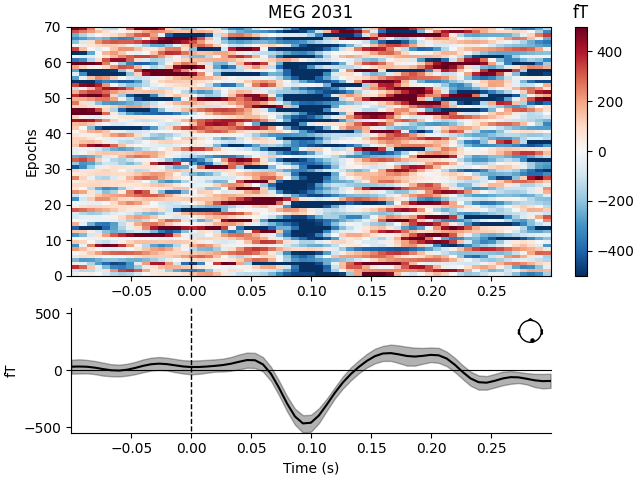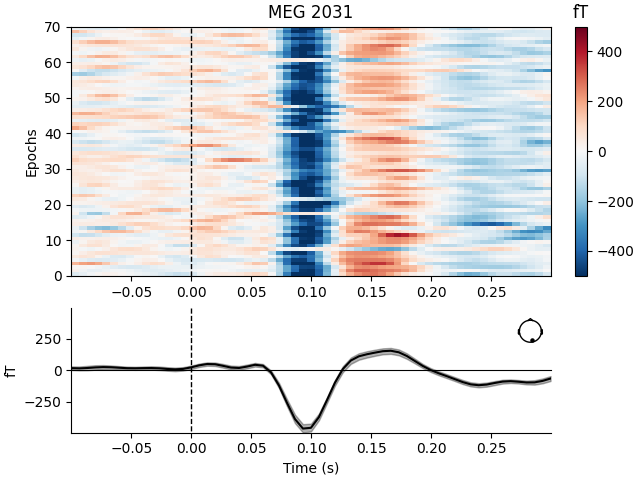# XDAWN Denoising#

XDAWN filters are trained from epochs, signal is projected in the sources space and then projected back in the sensor space using only the first two XDAWN components. The process is similar to an ICA, but is supervised in order to maximize the signal to signal + noise ratio of the evoked response .

Warning

As this denoising method exploits the known events to maximize SNR of the contrast between conditions it can lead to overfitting. To avoid a statistical analysis problem you should split epochs used in fit with the ones used in apply method.

```# Authors: Alexandre Barachant <alexandre.barachant@gmail.com>
#
```
```from mne import (io, compute_raw_covariance, read_events, pick_types, Epochs)
from mne.datasets import sample
from mne.preprocessing import Xdawn
from mne.viz import plot_epochs_image

print(__doc__)

data_path = sample.data_path()
```

```meg_path = data_path / 'MEG' / 'sample'
raw_fname = meg_path / 'sample_audvis_filt-0-40_raw.fif'
event_fname = meg_path / 'sample_audvis_filt-0-40_raw-eve.fif'
tmin, tmax = -0.1, 0.3
event_id = dict(vis_r=4)

# Setup for reading the raw data
raw.filter(1, 20, fir_design='firwin')  # replace baselining with high-pass

picks = pick_types(raw.info, meg=True, eeg=False, stim=False, eog=False,
# Epoching
epochs = Epochs(raw, events, event_id, tmin, tmax, proj=False,
verbose=False)

# Plot image epoch before xdawn
plot_epochs_image(epochs['vis_r'], picks=, vmin=-500, vmax=500)
``````Opening raw data file /home/circleci/mne_data/MNE-sample-data/MEG/sample/sample_audvis_filt-0-40_raw.fif...
Read a total of 4 projection items:
PCA-v1 (1 x 102)  idle
PCA-v2 (1 x 102)  idle
PCA-v3 (1 x 102)  idle
Average EEG reference (1 x 60)  idle
Range : 6450 ... 48149 =     42.956 ...   320.665 secs
Reading 0 ... 41699  =      0.000 ...   277.709 secs...
Filtering raw data in 1 contiguous segment
Setting up band-pass filter from 1 - 20 Hz

FIR filter parameters
---------------------
Designing a one-pass, zero-phase, non-causal bandpass filter:
- Windowed time-domain design (firwin) method
- Hamming window with 0.0194 passband ripple and 53 dB stopband attenuation
- Lower passband edge: 1.00
- Lower transition bandwidth: 1.00 Hz (-6 dB cutoff frequency: 0.50 Hz)
- Upper passband edge: 20.00 Hz
- Upper transition bandwidth: 5.00 Hz (-6 dB cutoff frequency: 22.50 Hz)
- Filter length: 497 samples (3.310 s)

[Parallel(n_jobs=1)]: Using backend SequentialBackend with 1 concurrent workers.
[Parallel(n_jobs=1)]: Done   1 out of   1 | elapsed:    0.0s remaining:    0.0s
[Parallel(n_jobs=1)]: Done   2 out of   2 | elapsed:    0.0s remaining:    0.0s
[Parallel(n_jobs=1)]: Done   3 out of   3 | elapsed:    0.0s remaining:    0.0s
[Parallel(n_jobs=1)]: Done   4 out of   4 | elapsed:    0.0s remaining:    0.0s
[Parallel(n_jobs=1)]: Done 366 out of 366 | elapsed:    0.6s finished
70 matching events found
No baseline correction applied
0 projection items activated
```

Now, we estimate a set of xDAWN filters for the epochs (which contain only the `vis_r` class).

```# Estimates signal covariance
signal_cov = compute_raw_covariance(raw, picks=picks)

# Xdawn instance
xd = Xdawn(n_components=2, signal_cov=signal_cov)

# Fit xdawn
xd.fit(epochs)
```
```Using up to 1388 segments
Number of samples used : 41640
[done]
Computing rank from data with rank='full'
MEG: rank 305 from info
Created an SSP operator (subspace dimension = 3)
Reducing data rank from 305 -> 305
Estimating covariance using EMPIRICAL
Done.
```

Epochs are denoised by calling `apply`, which by default keeps only the signal subspace corresponding to the first `n_components` specified in the `Xdawn` constructor above.

```epochs_denoised = xd.apply(epochs)

# Plot image epoch after Xdawn
plot_epochs_image(epochs_denoised['vis_r'], picks=, vmin=-500, vmax=500)
``````Transforming to Xdawn space
Zeroing out 303 Xdawn components
Inverse transforming to sensor space